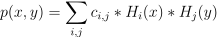/NumPy 1.17

# numpy.polynomial.hermite.hermval2d

numpy.polynomial.hermite.hermval2d(x, y, c) [source]

Evaluate a 2-D Hermite series at points (x, y).

This function returns the values:The parameters x and y are converted to arrays only if they are tuples or a lists, otherwise they are treated as a scalars and they must have the same shape after conversion. In either case, either x and y or their elements must support multiplication and addition both with themselves and with the elements of c.

If c is a 1-D array a one is implicitly appended to its shape to make it 2-D. The shape of the result will be c.shape[2:] + x.shape.

Parameters: x, y : array_like, compatible objects The two dimensional series is evaluated at the points (x, y), where x and y must have the same shape. If x or y is a list or tuple, it is first converted to an ndarray, otherwise it is left unchanged and if it isn’t an ndarray it is treated as a scalar. c : array_like Array of coefficients ordered so that the coefficient of the term of multi-degree i,j is contained in c[i,j]. If c has dimension greater than two the remaining indices enumerate multiple sets of coefficients. values : ndarray, compatible object The values of the two dimensional polynomial at points formed with pairs of corresponding values from x and y.

#### Notes

New in version 1.7.0.